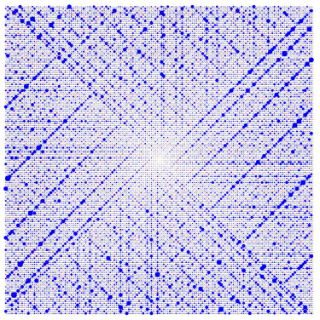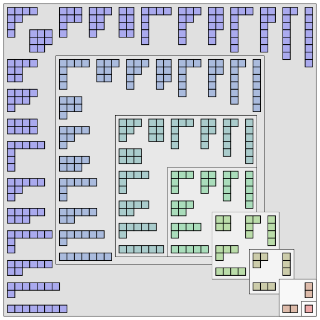# Number theory

Topic | v1 | created by jjones |Description

Number theory (or arithmetic or higher arithmetic in older usage) is a branch of pure mathematics devoted primarily to the study of the integers and integer-valued functions. German mathematician Carl Friedrich Gauss (1777–1855) said, "Mathematics is the queen of the sciences—and number theory is the queen of mathematics." Number theorists study prime numbers as well as the properties of mathematical objects made out of integers (for example, rational numbers) or defined as generalizations of the integers (for example, algebraic integers). Integers can be considered either in themselves or as solutions to equations (Diophantine geometry). Questions in number theory are often best understood through the study of analytical objects (for example, the Riemann zeta function) that encode properties of the integers, primes or other number-theoretic objects in some fashion (analytic number theory).

##### Relations

In number theory and combinatorics, a partition of a positive integer n, also called an integer parti...